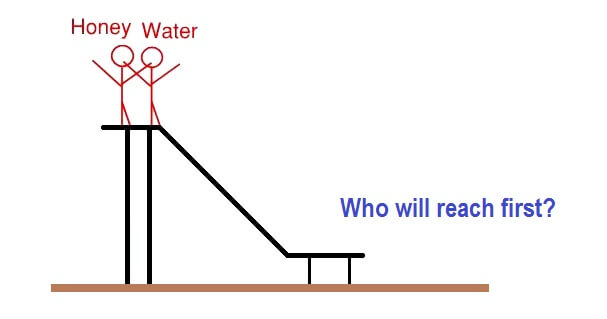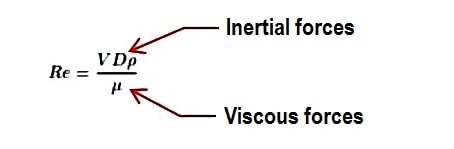# Why Viscosity is not included in the Orifice equation?

Has this question troubled you that viscosity is not included in the Orifice equation?

Imagine Honey flowing through the pipe and on the contrary water flowing through the Pipe will have the same pressure drop. You have guessed it right the pressure drop won’t be the same.

So why is viscosity not in equation? This question puzzled Tony Rudolph for 15 years until he had a Eureka moment.

## Demystifying Reynolds Number

We owe Sir Osborne Reynolds for this discovery.

To understand this let’s take a simple example.

Imagine we pour honey and water on a slide.

Who will reach first?Water will reach down first as compared to honey.

Why?

The answer in simple words is water has a lesser tendency to stick along its surface as compared to Honey.

This phenomenon is captured in Reynolds number.The “Numerator” consists of 3 variables that could be termed together as Inertial force.

V=Velocity, D=Diameter, p=density

While The “Denominator” consists of one term and that is absolute viscosity.

Hence, in conclusion, the Reynolds number is the ratio of “Inertial forces” to “Viscous force”.

So higher the Reynolds number the more “Inertial forces” dominate.

And for orifice meter one of the requirement is high Reynolds number.

Thus as “Viscous forces” at very high Reynolds are negligible we don’t consider them in the equation.

I hope it’s been of value to you.

Credits: I stumbled upon this insightful concept in Tony R Kuphaldt book “Lessons In Industrial Instrumentation”. A great read.